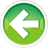# 4.4 — Signed integers

An integer is a integral type that can represent positive and negative whole numbers, including 0 (e.g. -2, -1, 0, 1, 2). C++ has 4 different fundamental integer types available for use:

Type Minimum Size Note
short 2 bytes
int 2 bytes Typically 4 bytes on modern architectures
long 4 bytes
long long 8 bytes C99/C++11 type

The key difference between the various integer types is that they have varying sizes -- the larger integers can hold bigger numbers.

A reminder

C++ only guarantees that integers will have a certain minimum size, not that they will have a specific size. See lesson 4.3 -- Object sizes and the sizeof operator for information on how to determine how large each type is on your machine.

Signed integers

By default, integers are signed, which means they can hold negative numbers. In this lesson, we’ll focus on signed integers. We’ll discuss unsigned integers (which can only hold non-negative numbers) in the next lesson.

Defining signed integers

Here is the preferred way to define the four types of signed integers:

All of the integers (except int) can take an optional int suffix:

This suffix should not be used. In addition to being more typing, adding the int suffix makes the type harder to distinguish from variables of type int. This can lead to mistakes if the short or long modifier is inadvertently missed.

The integer types can also take an optional signed keyword, which by convention is typically placed before the type name:

However, this keyword should not be used, as it is redundant, since integers are signed by default.

Best practice

Prefer the shorthand types that do not use the int suffix or signed prefix.

Signed integer ranges

As you learned in the last section, a variable with n bits can hold 2n different values. But which specific values? We call the set of specific values that a data type can hold its range. The range of an integer variable is determined by two factors: its size (in bits), and whether it is signed or not.

By definition, a 1-byte signed integer has a range of -128 to 127. This means a signed integer can store any integer value between -128 and 127 (inclusive) safely.

As an aside...

Math time: a 1-byte integer contains 8 bits. 28 is 256, so a 1-byte integer can hold 256 different values. There are 256 different values between -128 to 127, inclusive.

Here’s a table containing the range of signed integers of different sizes:

Size/Type Range
1 byte signed -128 to 127
2 byte signed -32,768 to 32,767
4 byte signed -2,147,483,648 to 2,147,483,647
8 byte signed -9,223,372,036,854,775,808 to 9,223,372,036,854,775,807

For the math inclined, an n-bit signed variable has a range of -(2n-1) to 2n-1-1.

For the non-math inclined… use the table. :)

Integer overflow

What happens if we try to assign the value 280 to a 1-byte signed integer? This number is outside the range that a 1-byte signed integer can hold. The number 280 requires 9 bits to represent, but we only have 8 bits available in a 1-byte integer.

Integer overflow (often called overflow for short) occurs when we try to store a value that is outside the range of the type. Essentially, the number we are trying to store requires more bits to represent than the object has available. In such a case, data is lost because the object doesn’t have enough memory to store everything.

In the case of signed integers, which bits are lost is not well defined, thus signed integer overflow leads to undefined behavior.

Warning

Signed integer overflow will result in undefined behavior.

In general, overflow results in information being lost, which is almost never desirable. If there is any suspicion that an object might need to store a value that falls outside its range, use a type with a bigger range!

Integer division

When dividing two integers, C++ works like you’d expect when the quotient is a whole number:

This produces the expected result:

```5
```

But let’s look at what happens when integer division causes a fractional result:

This produces a possibly unexpected result:

```1
```

When doing division with two integers (called integer division), C++ always produces an integer result. Since integers can’t hold fractional values, any fractional portion is simply dropped (not rounded!).

Taking a closer look at the above example, 8 / 5 produces the value 1.6. The fractional part (0.6) is dropped, and the result of 1 remains.

Similarly, -8 / 5 results in the value -1.

Warning

Be careful when using integer division, as you will lose any fractional parts of the quotient. However, if it’s what you want, integer division is safe to use, as the results are predictable.4.5 -- Unsigned integers, and why to avoid themIndex4.3 -- Object sizes and the sizeof operator

### 90 comments to 4.4 — Signed integers

•Amandeep

Why do we need to write "return 0;" in the last line of this program?

#include <iostream>

int main()
{
using namespace std;
unsigned short x = 0; // smallest 2-byte unsigned value possible
cout << "x was: " << x << endl;
x = x - 1; // overflow!
cout << "x is now: " << x << endl;
return 0;
}

•Todd

Typos.

"See lesson 23 (2.3) -- variable sizes and the sizeof operator"

"In lesson 21 (2.1) -- Basic addressing and variable declaration"

"If there is any doubt (suspicion) that a variable might need to store a value that falls outside its range, use a larger variable!" Either say 'suspicion that it won't work' or 'doubt that it will work', not 'doubt that it won't work' (double negative). You probably just started saying one these and switched when writing.

•Alex

Fixed! Many thanks.